## Geometry: 45º 45º 90º Special Triangles

45 45 90 triangle

Greetings math folks! In this post we are going to go over 45º 45º 90º special triangles and how to find the missing sides when given only one of its lengths. For even more examples, check out the video below and happy calculating! 🙂

## Why is it “special”?

The 45º 45º 90º triangle is special because it is an isosceles triangle, meaning it has two equal sides (marked in blue below).  If we know that the triangle has two equal lengths, we can find the value of the hypotenuse by using the Pythagorean Theorem.  Check it out below!

Now we can re-label our triangle, knowing the length of the hypotenuse in relation to the two equal legs. This creates a ratio that applies to all 45º 45º 90º triangles!

## How do I use this ratio?

Knowing the above ratio, allows us to find any length of a 45º 45º 90º triangle, when given the value of one of its sides.

Let’s try an example:

Now let’s look at an example where we are given the length of the hypotenuse and need to find the values of the other two missing sides.

Now try mastering the art of the 45º 45º 90º special triangle on your own!

## Solutions:

Still got questions? No problem! Don’t hesitate to comment with any questions or check out the video above. Happy calculating! 🙂

## Synthetic Division and Factoring Polynomials: Algebra 2/Trig.

Hey there math friends! In this post we will go over how and when to use synthetic division to factor polynomials! So far, in algebra we have gotten used to factoring polynomials with variables raised to the second power, but this post explores how to factor polynomials with variables raised to the third degree and beyond!

If you have any questions don’t hesitate to comment or check out the video below. Also, don’t forget to master your skills with the practice questions at the end of this post. Happy calculating! 🙂

## What is Synthetic Division?

Synthetic Division is a shortcut that allows us to easily divide polynomials as opposed to using the long division method. We can only use synthetic division when we divide a polynomial by a binomial in the form of (x-c), where c is a constant number.

## Example #1:

*Notice we can use synthetic division in this case because we are dividing by (x+4) which follows our parameters (x-c), where c is equal to 4.

## Example #2: Factoring Polynomials

Let’s take a look at the following example and use synthetic division to factor the given polynomial:

Check!

The great thing about these questions is that we can always check our work! If we wanted to check our answer, we could simply distribute 2(x+1)(x+3)(x-2) and get our original polynomial, f(x)=2x3+4x2-10x-12.

Try the practice problems on your own below!

Looking to brush up on how to divide polynomials the long way using long division? Check out this post here!

Still got questions? No problem! Don’t hesitate to comment with any questions or check out the video above. Happy calculating! 🙂

## Variance and Standard Deviation: Statistics

Greetings math friends! In this post we’re going to go over variance and standard deviation. We will take this step by step to and explain the significance each have when it comes to a set of data. Get your calculators ready because this step by step although not hard, will take some serious number crunching! Check out the video below to see how to check your work using a calculator and happy calculating! 🙂

What is the Variance?

The variance represents the spread of data or distance each data point is from the mean.  When we have multiple observations in our data, we want to know how far each unit of data is from the mean.  Are all the data points close together or spread far apart?  This is what the variance tells us!

Don’t freak out but here’s the formula for variance, notated as sigma squared:

This translates to:

Let’s try an example:

What is Standard Deviation?

Standard deviation is a unit of measurement that is unique to each data set and is used to measure the spread of data. The formula for standard deviation happens to be very similar to the variance formula!

Below is the formula for standard deviation, notated as sigma:

Since this is the same exact formula as variance with a square root, all we need to do is take the square root of the variance to find standard deviation:

Now try calculating these statistics on your own with the following practice problems!

Practice Questions:

Solutions:

Still got questions? No problem! Don’t hesitate to comment with any questions or check out the video above. Happy calculating! 🙂

Also! If you’re looking for more statistics, check out this post on how to create and analyze box and whisker plots here!

## Combining Like Terms and Distributive Property: Algebra

Greetings math peeps! In today’s post, we are going to review some of the basics: combining like terms and distributive property. It’s so important to master the basics such as these, so you’re prepared and ready to handle the harder stuff that’s just around the corner, trust me they’re coming! And for those who already feel comfortable with these topics, great! Skip ahead and try the practice questions at the bottom of this post and happy calculating! 🙂

## When do we combine “like terms?”

Combining like terms allows us to simplify and calculate our answer with terms that have the same variable and same exponent values only. For example, we can combine the following expression:

## How do we combine like terms?

We add or subtract the whole number coefficients and keep the variable they have in common.

Why? We could not add these two terms together because their variables do not match! 2 is multiplied by x, while 3 is multiplied by the variable xy.

Why? We could not add these two terms together because their variables and exponents do not match! 2 is multiplied by x, while 3 is multiplied by the variable x^2. Exponents for each variable must match to be considered like terms.

## Distributive Property:

Combining like terms and the distributive property go hand in hand.  The distributive property rule states the following:

There are no like terms to combine in the example above, but let’s see what it would like to use the distributive property and combine like terms at the same time with the following examples:

## Example #2:

In some cases, we also have to distribute the-1 that can sometimes “hide” behind a parenthesis.

Try the following questions on your own on combining like terms and the distributive property and check out the video above for more!

## Solutions:

Still got questions? No problem! Don’t hesitate to comment with any questions or check out the video above. Happy calculating! 🙂

Looking to review more of the basics? Check out this post on graphing equations of a line y=mx+b here.

## Transversals and Parallel Lines: Geometry

Happy Wednesday math friends! In this post we are going to look at transversals and parallel lines and find the oh so many congruent and supplementary angles they form when they come together! Congruent angles that form with these types of lines are more commonly known as Alternate Interior Angles, Alternate Exterior Angles, Corresponding angles, and Supplementary angles. Let’s look at this one step at a time:

## What are Transversals and Parallel Lines?

When two parallel lines are cut by a diagonal line ( called a transversal) it looks something like this:

Each angle above has at least one congruent counterpart. There are several different types of congruent relationships that happen when a transversal cuts two parallel lines and we are going to break each down:

## 1) Alternate Interior Angles:

When a transversal line cuts across two parallel lines, opposite interior angles are congruent.

## 2) Alternate Exterior Angles:

When a transversal line cuts across two parallel lines, opposite exterior angles are congruent.

## 3) Corresponding Angles:

When a transversal line cuts across two parallel lines, corresponding angles are congruent.

## 4) Supplementary Angles:

Supplementary angles are a pair of angles that add to180 degrees. 180 degrees is the value of distance found within a straight line, which is why you’ll find so many supplementary angles below:

Knowing the different sets of congruent and supplementary angles, we can easily find any missing angle values when faced with the following question:

-> Using our knowledge of congruent and supplementary angles we should be able to figure this out! Right away we can find angle 2 by noticing angle 1 and angle 2 are supplementary angles (add to 180 degrees).-> Knowing angle 2 is50 degrees, we can now fill in the rest of our transversal angles based on our corresponding and supplementary rules.

Try the following transversal and parallel lines questions below! Some may a bit harder than the previous example, if you get stuck, check out the video that goes over a similar example above and happy calculating! 🙂

Practice Questions:

1. Find the value of the missing angles given line ris parallel to lines and line t is a transversal.

2. Find the value of the missing angles given liner is parallel to lines and line t is a transversal.

Solutions:

Still got questions? No problem! Don’t hesitate to comment with any questions or check out the video above. Happy calculating! 🙂

Also, if you’re looking to learn about the difference between parallel and perpendicular lines, check out this post here!

## Imaginary and Complex Numbers: Algebra 2/Trig.

Happy Wednesday and back to school season math friends! This post introduces imaginary and complex numbers when raised to any power exponent and when multiplied together as a binomial. When it comes to all types of learners, we got you between the video, blog post, and practice problems below. Happy calculating! 🙂

What are Imaginary Numbers?

Imaginary numbers happen when there is a negative under a radical and looks something like this:

Why does this work?

In math, we cannot have a negative under a radical because the number under the square root represents a number times itself, which will always give us a positive number.

Example:

But wait, there’s more:

When raised to a power, imaginary numbers can have the following different values:

Knowing these rules, we can evaluate imaginary numbers, that are raised to any value exponent! Take a look below:

-> We use long division, and divide our exponent value 54, by 4.

-> Now take the value of the remainder, which is 2, and replace our original exponent. Then evaluate the new value of the exponent based on our rules.

What are Complex Numbers?

Complex numbers combine imaginary numbers and real numbers within one expression in a+bi form. For example, (3+2i) is a complex number. Let’s evaluate a binomial multiplying two complex numbers together and see what happens:

-> There are several ways to multiply these complex numbers together. To make it easy, I’m going to show the Box method below:

Try mastering imaginary and complex numbers on your own with the questions below!

Practice:

Solutions:

Still got questions? No problem! Don’t hesitate to comment with any questions or check out the video above. Don’t forget to sign up for FREE weekly MathSux videos, lessons, and practice questions. Thanks for stopping by and happy calculating! 🙂

Also, if you’re looking to learn more about dividing polynomials, check out this post here!

## Box and Whisker Plots, IQR and Outliers: Statistics

Ahoy math friends! This post takes a look at one method of analyzing data; box and whisker plots. Box and whisker plots are great for visually identifying outliers and the overall spread of numbers in a data set. We will go over step by step how to create a box and whisker plot given a set of data, we will then look at how to find the interquartile range and upper and lower outliers. If you have any questions, don’t hesitate to check out the video or comment below. Stay curious and happy calculating! 🙂

Looking for more MathSux? Check out this post on variance and standard deviation here!

Box plots look something like this:

Why Box Plots?

Box Plots are a great way to visually see the distribution of a set of data.  For example, if we wanted to visualize the wide range of temperatures found in a day in NYC, we would get all of our data (temperatures for the day), and once a box plot was made, we could easily identify the highest and lowest temperatures in relation to its median (median: aka middle number).

From looking at a Box Plot we can also quickly find the Interquartile Range and upper and lower Outliers. Don’t worry,  we’ll go over each of these later, but first, let’s construct our Box Plot!

->  First, we want to put all of our temperatures in order from smallest to largest.
-> Now we can find Quartile 1 (Q1), Quartile 2 (Q2) (which is also the median), and Quartile 3 (Q3).  We do this by splitting the data into sections and finding the middle value of each section.

Q1=Median of first half of data

Q2=Median of entire data set

Q3=Median of second half of data

-> Now that we have all of our quartiles, we can make our Box Plot! Something we also have to take notice of, is the minimum and maximum values of our data, which are 65 and 92 respectively. Let’s lay out all of our data below and then build our box plot:

Now that we have our Box Plot, we can easily find the Interquartile Range and upper/lower Outliers.

->The Interquartile Range is the difference between Q3 and Q1. Since we know both of these values, this should be easy!

Next, we calculate the upper/lower Outliers.

-> The Upper/Lower Outliers are extreme data points that can skew the data affecting the distribution and our impression of the numbers. To see if there are any outliers in our data we use the following formulas for extreme data points below and above the central data points.

*These numbers tell us if there are any data points below 44.75 or above 114.75, these temperatures would be considered outliers, ultimately skewing our data. For example, if we had a temperature of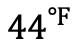or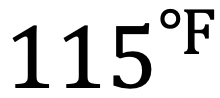these would both be considered outliers.

Practice Questions:

Solutions:

Still got questions? No problem! Don’t hesitate to comment with any questions or check out the video above. Happy calculating! 🙂

## How to Construct an Equilateral Triangle?: Geometry

Happy Wednesday math peeps! This post introduces constructions by showing us how to construct an equilateral triangle by using a compass and a ruler. For anyone new to constructions, this is the perfect topic for art aficionados since there is more drawing than there is actual math.

## What is an Equilateral Triangle?

Equilateral Triangle: A triangle with three equal sides.  Not an easy one to forget, the equilateral triangle is super easy to construct given the right tools (compass+ ruler). Take a look below:

Now, for our Example:

## What’s Happening in this GIF?

1. Using a compass, measure out the distance of line segment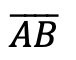.

2. With the compass on point A, draw an arc that has the same distance as.

3. With the compass on point B, draw an arc that has the same distance as.

4. Notice where the arcs intersect? Using a ruler, connect points A and B to the new point of intersection. This will create two new equal sides of our triangle!

Still got questions? No problem! Don’t hesitate to comment with any questions. Happy calculating! 🙂

Looking to learn more about triangles? Check out this post on right triangle trigonometry here!

## Expanding Cubed Binomials: Algebra 2/Trig.

Greetings math friends! This post will go over expanding cubed binomials using two different methods to get the same answer. We’re so used to seeing squared binomials such as,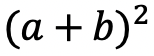, and expanding them without a second thought.  But what happens when our reliable squared binomials are now raised to the third power, such as,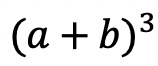?  Luckily for us, there is a Rule we can use:

But where did this rule come from?  And how can we so blindly trust it? In this post we will prove why the above rule works for expanding cubed binomials using 2 different methods:

Why bother? Proving this rule will allow us to expand and simplify any cubic binomial given to us in the future! And since we are proving it 2 different ways, you can choose the method that best works for you.

## Method #1: The Box Method

Step 1: First, focus on the left side of the equation by expanding (a+b)3:

Step 2: Now we are going to create our first box, multiplying (a+b)(a+b). Notice we put each term of (a+b) on either side of the box. Then multiplied each term where they meet.

Step 3: Combine like terms ab and ab, then add each term together to get a2+2ab+b2.

Step 4: Multiply (a2+2ab+b2)(a+b) making a bigger box to include each term.

Step 5: Now combine like terms (2a2b and a2b) and (2ab2 and ab2), then add each term together and get our answer: a3+3a2b+3ab2+b3.

## Method #2: The Distribution Method

Let’s expand the cubed binomial using the distribution method step by step below:

Now that we’ve gone over 2 different methods of cubic binomial expansion, try the following practice questions on your own using your favorite method!

Practice Questions: Expand and simplify the following.

Solutions:

Still, got questions?  No problem! Check out the video above or comment below! Happy calculating! 🙂

**Bonus: Test your skills with this Regents question on!

## How to use Recursive Formulas?: Algebra

Welcome to Mathsux! This post is going to show you everything you need to know about Recursive Formulas by looking at three different examples. Check out the video below for more of an explanation and test your skills with the practice questions at the bottom of this page.  Happy calculating! 🙂

## What is a Recursive Formula?

A Recursive Formula is a type of formula that forms a sequence based on the previous term value.  What does that mean?  Check out the example below for a clearer picture:

## Example #1:

-> First, let’s decode what these formulas are saying.

-> We found the sequence 2, 6, 10, 14, 18. Since we only needed the fifth term to answer our question, we know our solution is 18.

## Example #2:

-> First, let’s decode what these formulas are saying.

***Note this was written in a different notation but is solved in the exact same way!

-> We found the sequence 1,3,9. Since we only needed to find the third term to answer our questions, we know our solution is 9.

-> First, let’s decode what these formulas are saying.

-> We found the sequence 4,10, 28. Since we only needed to find the third term to answer our question, we know the solution is 28.

## Solutions:

Still got questions? No problem! Check out the video above for more or try the NYS Regents question below, and please don’t hesitate to comment with any questions. Happy calculating! 🙂

***Bonus! Want to test yourself with a similar NYS Regents question on Recursive Formulas?  Click here!